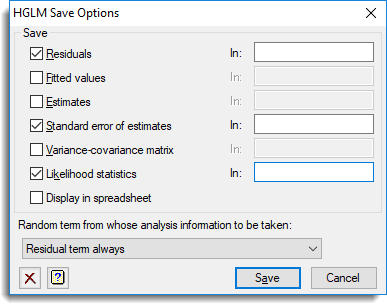1. Home
2. HGLM Save Options

# HGLM Save Options

Use this to save results from an HGLM analysis in Genstat data structures.

1. After selecting the appropriate boxes, type the identifiers of the data structures into the corresponding In: fields.## Save

 Residuals Variate Deviance residuals from the analysis Fitted values Variate Fitted values from the analysis Estimates Variate Estimates of parameters Standard error of estimates Variate Standard errors of the estimates Variance-covariance matrix Symmetric matrix Variance-covariance matrix of each set of estimates Likelihood statistics Variate Likelihood statistics from the fitted model. There are 8 statistics saved: -2*h(y|v), -2*h, -2*P_v(h), -2*P_beta,v(h), -2*EQD(y|v), -2*EQD, -2*P_v(EQD) and -2*P_beta,v(EQD), where EQD = extended quasi-deviance. These statistics are explained on page 288 of the Statistics Guide.

The saved results will be displayed in a new spreadsheet.

## Random terms from whose analysis information to be taken

This provides a list of random terms from whose analysis the information is to be saved. If you want to save the information from the full model then select the Residual term from full model option from the list.